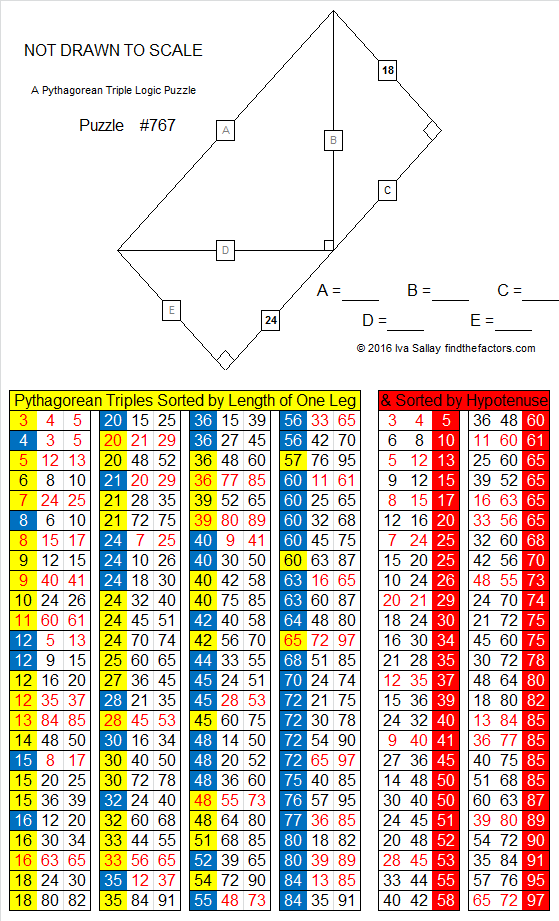# 767 is the hypotenuse of a Pythagorean triple, but…

• 767 is a composite number.
• Prime factorization: 767 = 13 x 59
• The exponents in the prime factorization are 1 and 1. Adding one to each and multiplying we get (1 + 1)(1 + 1) = 2 x 2 = 4. Therefore 767 has exactly 4 factors.
• Factors of 767: 1, 13, 59, 767
• Factor pairs: 767 = 1 x 767 or 13 x 59
• 767 has no square factors that allow its square root to be simplified. √767 ≈ 27.69476.Because 13 is one of its factors, 767 is the hypotenuse of Pythagorean triple 295-708-767, but 767 will never be part of the solution to one of these Pythagorean triple puzzles because it is greater than 100. Every side and hypotenuse in these puzzles must be a whole number less than 100. Together the numbers in the puzzle must form Pythagorean triples. Can you solve the puzzle?Print the puzzles or type the solution on this excel file: 12 Factors 2016-02-25

————————————-

Here’s a little more about the number 767:

767 is a palindrome in two different bases:

• 767 in BASE 10; note that 7(100) + 6(10) + 7(7) = 767.
• 2B2 in BASE 17 (B = 11 base 10); note that 2(289) + 11(17) + 2(1) = 767.

Wikipedia informs us that 767 is also the 9th Thabit number.

————————————-